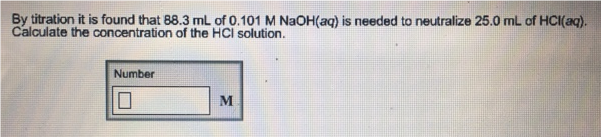# Problem: By titration it is found that 88.3 mL of 0.101 M NaOH(aq) is needed to neutralize 25.0 mL of HCl(aq). Calculate the concentration of the HCl solution.

###### FREE Expert Solution
99% (255 ratings)###### Problem Details

By titration it is found that 88.3 mL of 0.101 M NaOH(aq) is needed to neutralize 25.0 mL of HCl(aq). Calculate the concentration of the HCl solution.